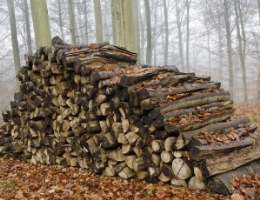Calculating wood store capacity for a biomass boilerImage: dan / FreeDigitalPhotos.net

# Wood Fuel Store

Fuel storage facilities normally account for a significant proportion of the overall capital cost of biomass heating system. It is important to size fuel storage on the site heat demand and demand profile.

Biomass Boiler Wood Storage Volume

To estimate the required storage capacity of wood chips or wood pellets for a ´domestic´ sized boiler, follow this simple rule of thumb. It assumes a 8 week supply§, equivalent to 4 weeks at full operation and 4 weeks at 50% load.

• 8 hours operation x 5 days a week x 4 weeks x peak load (of boiler) x boiler efficiency = Energy used (kWh)
•   --  plus  --
• 8 hours operation x 5 days a week x 4 weeks x half load (of boiler) x boiler efficiency = Energy used (kWh)
•   --  adjust the hours and days according to use  --
• next divide the energy used by the heat content (CV) of the wood per kg taking account of the boiler efficiency
• so, energy used in period kWh /CV (kWh/kg) x boiler efficiency (say 0.9) = wood mass volume (kg)
• divide this figure by the bulk volume to get storage volume
• wood mass volume (kg)/ bulk volume (kg/m3) = minimum storage volume (m3)

§Note a 8 week supply with 3 winter fills and 1 summer fill should cover the whole heating season - 32 weeks supply which is approximately equivalent to the annual heating period

### Example Wood Store Size

The storage for a 60kW boiler using pellets would be as follows:

• (8 x 5 x 4 x 60 x 0.9)+ (8 x 5 x 4 x 30 x 0.9) = 8,640 + 4,320 = 12,960 kWh
• so, 12,960 / 4.4 = 2,945 kg of pellets
• therefore the minimum volume for pellet storage is 2,945 / 650 = 4.5 m3
• volume for wood chip storage is(12,960 / 3.1) / 230 = 18.1 m3

Where wood has the following properties:

 CV Bulk Density Wood Chip 3.1 kWh/kg 230 kg/m3 Pellets 4.4 kWh/kg 650 kg/m3

The storage volume should be at least twice the delivery volume.

Wood Storage Volume - last updated 30th January, 2014 by Corny